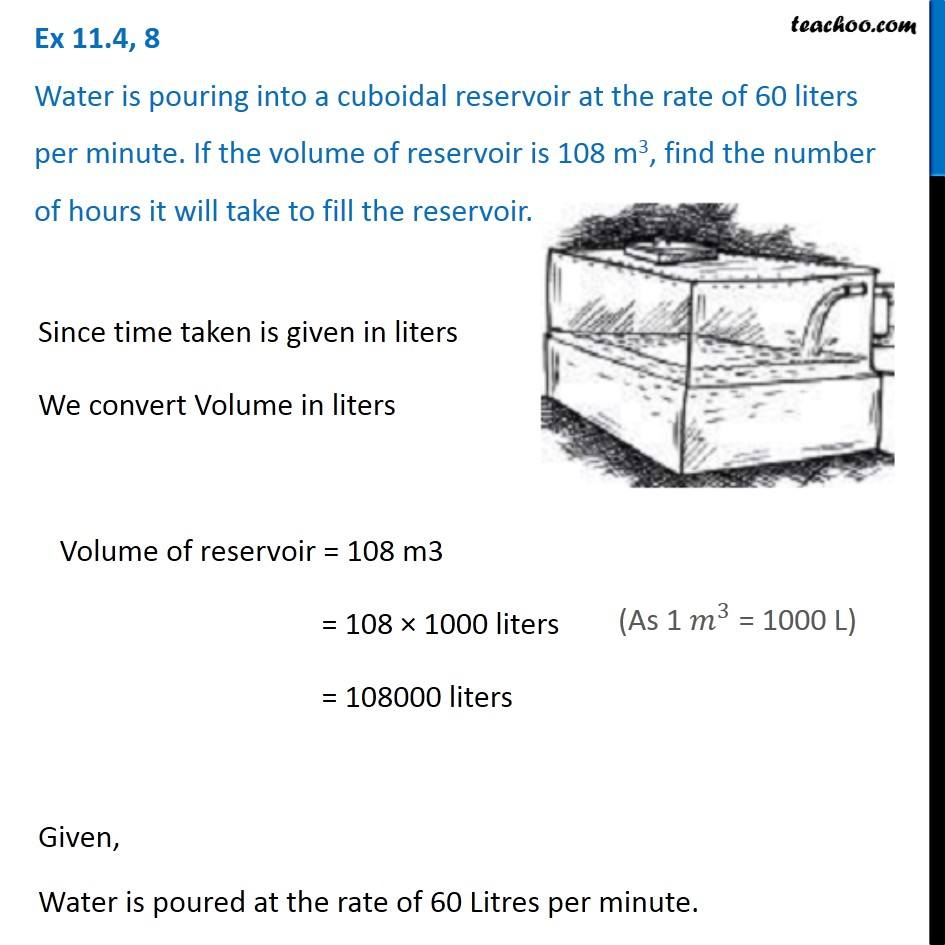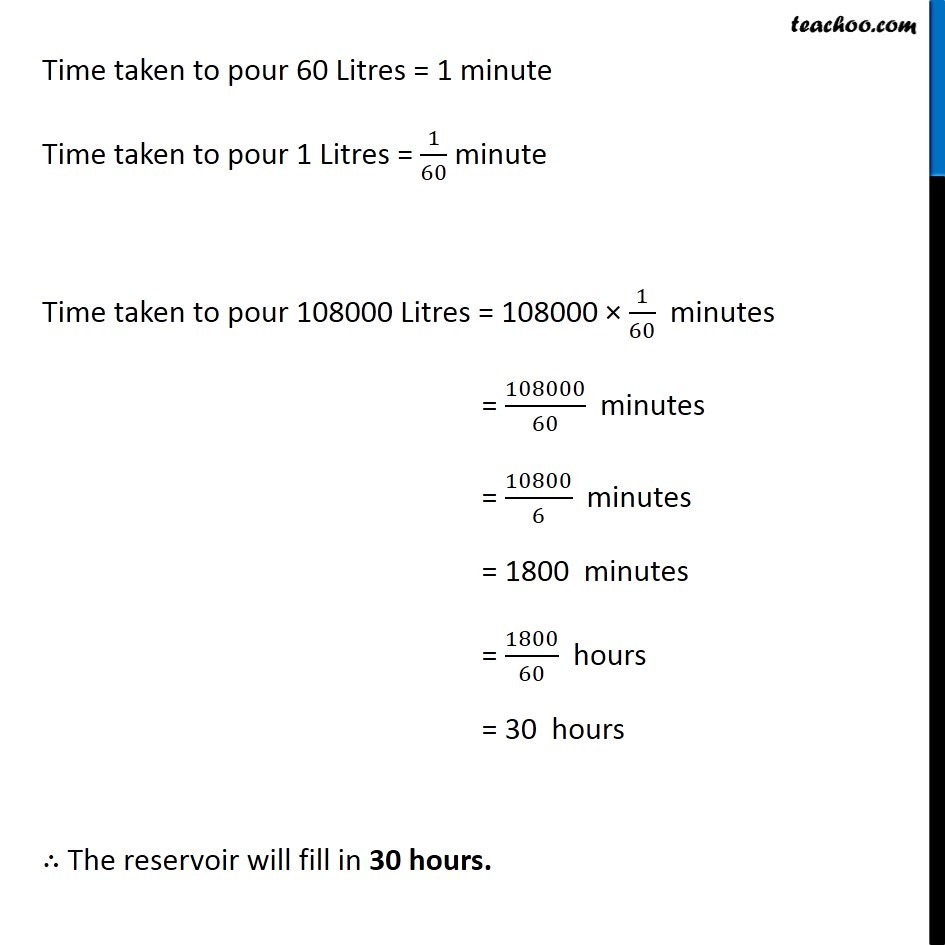1. Chapter 11 Class 8 Mensuration
2. Serial order wise
3. Ex 11.4

Transcript

Ex 11.4, 8 Water is pouring into a cuboidal reservoir at the rate of 60 liters per minute. If the volume of reservoir is 108 m3, find the number of hours it will take to fill the reservoir. Since time taken is given in liters We convert Volume in liters Volume of reservoir = 108 m3 = 108 × 1000 liters = 108000 liters Given, Water is poured at the rate of 60 Litres per minute. Time taken to pour 60 Litres = 1 minute Time taken to pour 1 Litres = 1/60 minute Time taken to pour 108000 Litres = 108000 × 1/60 minutes = 108000/60 minutes = 10800/6 minutes = 1800 minutes = 1800/60 hours = 30 hours ∴ The reservoir will fill in 30 hours.

Ex 11.4

Chapter 11 Class 8 Mensuration
Serial order wise